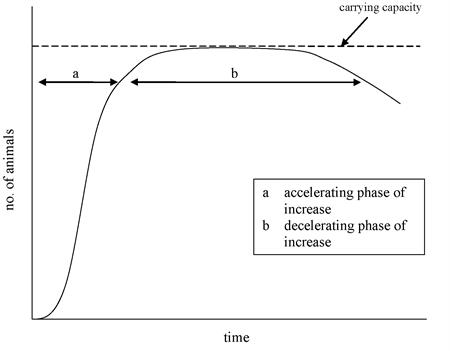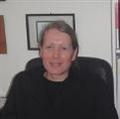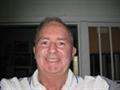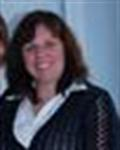# Statistics

Online course in statistics & statistical methods, learn data collection and processing, determining trends, calculations, distributions central tendency.

Course Code BSC304 S3 100 hours Statement of Attainment

 Tweet

## It's Easy to Enrol

Select a Learning Method

I am studying from...

Enable Javascript to automatically update prices.

All prices in Australian Dollars.

Click on Enrol Now to See Our Payment Plans Available. No Obligation.

Courses can be started at any time from anywhere in the world!Rise above the mean with statistics!

Strengthen your career with this course in statistics. If you want to develop scientifically-based research studies, this is your starting point. Learn how to interpret data sets, and how to prove your point based on scientifically proven methods

This course provides the most essential knowledge and skills required by consultants and researchers in a wide variety of disciplines. This course assumes a basic knowledge of Statistics, at least to the level covered by our Research Project I course.

## Lesson Structure

There are 10 lessons in this course:

1. Introduction
• Key terms and concepts: data, variables
• Measurements of scale: nominal, ordinal, interval,ratio
• Data presentation
• Probability
• Rounding of data
• Scientific notation
• Significant figures
• Functions
• Equations
• Inequalities
• Experimental design
• The normal curve
• Data collection
• Simple, systemic, stratified and cluster random sampling
• Remaining motivated to learn statistics
2. Distributions
• Scope and nature of distributions
• Class intervals and limits
• Class boundaries
• Frequency Distribution
• Histograms
• Frequency polygons
• Normal distributions
• Other distributions
• Frequency curves
3. Measures of central tendency
• Range, percentiles, quartiles, mode, median, mean
• Variance
• Standard deviation
• Degrees of freedom
• Interquartile and semi interquartile deviations
4. The Normal curve and Percentiles and Standard Scores
• Normal distribution characteristics
• Percentiles
• Standard scores
• Z scores
• T score
• Converting standard scores to percentiles
• Area under a curve
• Tables of normal distribution
5. Correlation
• Scope and nature of Correlation
• Correlation coefficient
• Cooeficient of determination
• Scatter plots
• Product movement forlinear correlation coefficient
• Rank correlation
• Multiple correlation
6. Regression
• Calculating regression equation with correlation coefficient
• Least squares method
• Standard error of the estimate
7. Inferential Statistics
• Hypothesis testing
• Test for a mean
• Errors in accepting or rejecting null hypothesis
• Levels of significance
• One and two tailed tests
• Sampling theory
• Confidence intervals
8. The t Test
• Assessing statistical difference with the t test
• t Test for independant samples
• t Test for dependant (paired) samples
9. Analysis of variance
• Scope and application of ANOVA
• Factors and levels
• Hypothesis
• Calculate degrees of freedon
• Calculate sum of squares within and between groups
• Calculate mean square
• Calculate F
10. Chi square test
• Chi quare goodness of fit test
• Calculate degrees of freedom
• Chi square test of independance
• Calculate expected frquencies
• Degrees of freedon
• Contingency tables
• Find expected frequencies
• Calculate degrees of freedom

Each lesson culminates in an assignment which is submitted to the school, marked by the school's tutors and returned to you with any relevant suggestions, comments, and if necessary, extra reading.

## Aims

• Become familiar with different statistical terms and the elementary representation of statistical data.
• Become familia with distributions, and the application of distributions in processing data.
• Apply measures of central tendency in solving research questions
• Demonstrate and explain the normal curve, percentiles and standard scores.
• Explain methods of correlation that describes the relationship between two variables.
• Make predictions with regression equations.
• Determine how much error to expect when making the predictions.
• Explain the basic concepts of underlying the use of statistics to make inferences.
• Analyze the difference between the means of two groups with the t Test.
• Describe the use of ANOVA (Analysis of Variance) in analysing the difference between two or more groups.
• Apply the concept of Non Parametric Statistics

### There are different types of statistics.

Descriptive statistics describe a set of data, while inferential statistics make inferences about large groups based on data from a smaller subset of the group.  To infer means to draw a conclusion based on facts or premises.  Thus an inference is the end result; a proposition based on the act of inferring.

Understanding how to gather and analyze statistics is the starting point for using statistical data in real world situations, to make better decisions for planning and management, at work, play or in any other aspect of your life.

When you comprehend these things, you will understand how statistics is something that can make your life better, wherever you live, and whatever you do.

This course will introduce you to the science behind statistics - there is a growing need for data science experts today. Almost all businesses and industries use statistical data to see how their businesses are trending and how business can be made to grow by analysing trends and directing business policy and decisions.

The role of the data scientist is to analyse trends, predict future directions and use this statistical analysis to suggest better ways to improve and grow businesses.

## MAKE THE BEST DECISION

Let us help with our Free Career and Course Counselling Service.

Contact one of our science academics.
Learn from our experience.

or Enrol
-go to top of the page for enrolment (right column)

## Start Now!Christine Todd

University lecturer, businesswoman, photographer, consultant and sustainability expert; with over 40 years industry experience B.A., M.Plan.Prac., M.A.(Social). An expert in planning, with years of practical experience in permaculture.Jacinda Cole

Psychologist, Educator, Author, Psychotherapist. B.Sc., Psych.Cert., M. Psych. Cert.Garden Design, MACA Jacinda has over 25 years of experience in psychology, in both Australia and England. She holds a BSc (Hons) in Psychology and a Masters in PsycholoRobert James

B.App. Sc. (Horticulture), Dip.Ag., M.Sc., Grad Dip.Mgt. Over 50 years experience that includes, Nursery Manager Brisbane City Councoil, Grounds Manager (University of Qld), Lecturer Qld Agricultural College, Propagator/Nurseryman at Aspley Nursery, HortTracey Jones

B.Sc. (Psych), M.Soc.Sc., Dip.Social Work, P.G.Dip Learning Disability, Cert Editing, Cert Creative Writing, PGCE. Member British Psychological Society, Member Assoc. for Coaching, Member British Learning Assoc. 25 years industry experience in writing,